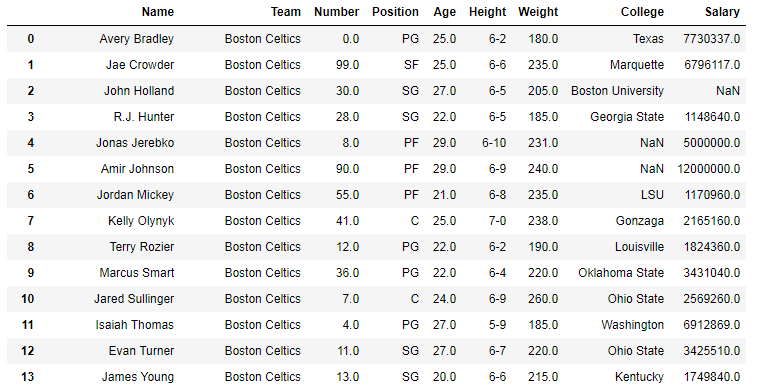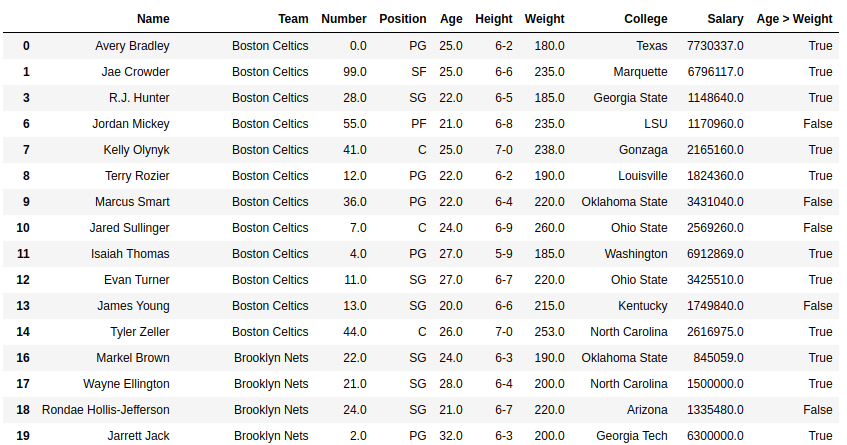Related Articles

# Python | Pandas Series.gt()

• Last Updated : 06 Jan, 2020

Python is a great language for doing data analysis, primarily because of the fantastic ecosystem of data-centric Python packages. Pandas is one of those packages and makes importing and analyzing data much easier.

Pandas `Series.gt()` is used to compare two series and return Boolean value for every respective element.

Syntax: Series.gt(other, level=None, fill_value=None, axis=0)

Parameters:
other: other series to be compared with
level: int or name of level in case of multi level
fill_value: Value to be replaced instead of NaN
axis: 0 or ‘index’ to apply method by rows and 1 or ‘columns’ to apply by columns.

Return type: Boolean series

Note: The results are returned on the basis of comparison caller series > other series.

In the following examples, the data frame used contains data of some NBA players. The image of data frame before any operations is attached below.Example #1:
In this example, the Age column and Weight columns are copared using .gt() method. Since values in weight columns are very large as compared to Age column, hence the values are divided by 10 first. Before comparing, Null rows are removed using .dropna() method to avoid errors.

 `# importing pandas module ``import` `pandas as pd `` ` `# importing regex module``import` `re``   ` `# making data frame ``data ``=` `pd.read_csv(``"https://media.geeksforgeeks.org/wp-content/uploads/nba.csv"``) ``   ` `# removing null values to avoid errors ``data.dropna(inplace ``=` `True``) `` ` `# other series``other ``=` `data[``"Weight"``]``/``10`` ` `# calling method and returning to new column``data[``"Age > Weight"``]``=` `data[``"Age"``].gt(other)`

Output:
As shown in the output image, the new column has True wherever value in Age column is greater than Weight/10.Example #2: Handling NaN values

In this example, two series are created using `pd.Series()`. The series contains null value too and hence 5 is passed to fill_value parameter to replace null values by 5.

 `# importing pandas module ``import` `pandas as pd `` ` `# importing numpy module``import` `numpy as np`` ` `# creating series 1``series1 ``=` `pd.Series([``24``, ``19``, ``2``, ``33``, ``49``, ``7``, np.nan, ``10``, np.nan])`` ` `# creating series 2``series2 ``=` `pd.Series([``16``, np.nan, ``2``, ``23``, ``5``, ``40``, np.nan, ``0``, ``9``])`` ` `# setting null replacement value``na_replace ``=` `5`` ` `# calling and storing result``result ``=` `series1.gt(series2, fill_value ``=` `na_replace)`` ` `# display``result`

Output:
As it can be seen in output, NaN values were replaced by 5 and the comparison is performed after the replacement and new values are used for comparison.

```0     True
1     True
2    False
3     True
4     True
5    False
6    False
7     True
8    False
dtype: bool
```

Attention geek! Strengthen your foundations with the Python Programming Foundation Course and learn the basics.

To begin with, your interview preparations Enhance your Data Structures concepts with the Python DS Course. And to begin with your Machine Learning Journey, join the Machine Learning – Basic Level Course

My Personal Notes arrow_drop_up